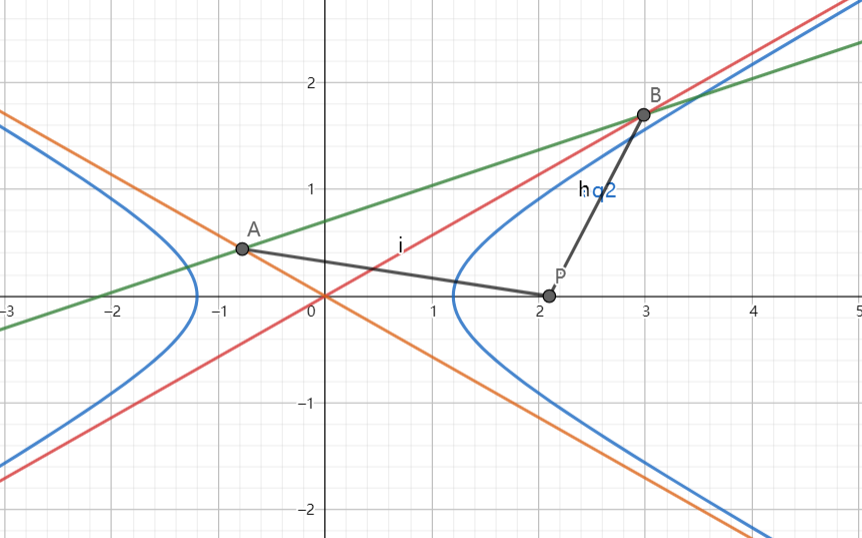# SAT1000 - P759

Geometry Level 2As shown above, the line: $x-3y+m=0\ (m \neq 0)$ intersects with the two asymptotes of the hyperbola:

$\dfrac{x^2}{a^2}-\dfrac{y^2}{b^2}=1\ (a>0,b>0)$ at point $A,B$.

If point $P$ is at $(m,0)$ and $|PA|=|PB|$, find the eccentricity of the hyperbola.

Let $E$ denote the eccentricity, submit $\lfloor 1000E \rfloor$.

Have a look at my problem set: SAT 1000 problems

×